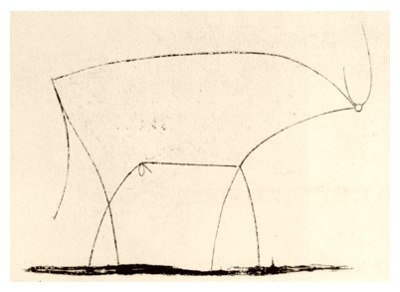# Precise AbstractionsArt lover will probably recognize the image above. It depicts the absolute essence of a bull and Picasso named it "Bull - plate 11". It is an abstraction. Note the number 11 in the title. It is the eleventh image in a series, which starts with a more lifelike bull.

When programming, have you ever used an abstraction? Sure you did. Have you ever abstracted something yourself? Sure you did. Abstraction is one of the core techniques for all programmers. So, why do we do it?

Abstracting has a psychological aspect. We cannot keep that many concepts in mind, but we want to design and implement hugely complex systems with our computers. What our minds do is chunking: Clump a bunch of things together into a chunk, such that it is only one thing. We must abstract to make complexity feasible.

A long long time ago when the assembly programmers roamed the earth, they invented the subroutine. That is a really useful abstraction in assembly. Then god gave us high-level languages like C, where we have function calls. Here is a simple function, which computes the greatest common divisor of two integers using Euclid's algorithm.

```int gcd(int a, int b) {
while (n != 0) {
int t = m % n;
m = n;
n = t;
}
return m
}
```

There is a faster algorithm, but that one is longer. For the sake of simplicity, we use this one.

Being abstract is something profoundly different from being vague … The purpose of abstraction is not to be vague, but to create a new semantic level in which one can be absolutely precise. –E. W. Dijkstra

Let us apply Dijkstra's insight. The perfect abstraction of gcd should absolute precisely apply to everything it can be applied to. This gcd C function is vague in a limiting sense. It is limited to int numbers. In C++ you could write a template function, which works for double and other numbers as well. In fact, anything which has a zero element and a modulo operation.

```template <R>
R gcd(R m, R n) {
while (n != R(0)) {
R t = m % n;
m = n;
n = t;
}
return m;
}
```

You see that the function signature has changed and we wrapped the zero. The algorithm looks the same. You can use this version for anything, but it is not precise. Unfortunately, it can be applied to values where it makes no sense. For example, if you apply it to strings the compiler will complain. However, there are types with zero and modulo, but this algorithm will not compute the greatest common divisor. There are even types with zero and modulo, where there is no greatest common divisor. The compiler will not complain and the program just computes something wrong. This particular algorithm computes gcd for types in the euclidean domain. Could we write the following?

```template <EuclideanDomain R>
R gcd(R m, R n) {
while (n != R(0)) {
R t = m % n;
m = n;
n = t;
}
return m;
}
```

This code is from Stepanov's Journeys. If you are interested in more math around gcd, read that.

Stepanov uses the proposed C++ feature concepts. Concepts were proposed for C++11, but ultimately rejected. It evolved into "Concepts-Lite", which failed to be included in C++17. Instead we now switch to D. The syntax slightly changes. Instead of foo<bar>, you write foo!(bar). So, we can write the algorithm like this:

```R gcd(R)(R m, R n) {
static assert (isEuclideanDomain!(R));
while (n != R(0)) {
R t = m % n;
m = n;
n = t;
}
return m;
}
```

The problem with the approach above? What if your type is not an euclidean domain? Stein's algorithm works on things, where Euclid's does not. Anyways, we want to be open for future extension. Here is slightly different variant:

```R gcd(R)(R m, R n) if (isEuclideanDomain!R) {
while (n != R(0)) {
R t = m % n;
m = n;
n = t;
}
return m;
}
```

If you try to use a type, which fails the isEuclideanDomain check, the compiler will complain that there is no matching function. Now what happens if you define a second gcd function with a different check? The compiler will try both and pick the one that fits or complain about ambiguity if both checks succeed.

This function finally fulfills Dijkstra's demand to be absolutely precise about where it can be applied. Like Picasso, it did take us a few iterations to get there.

Update, thanks to Chris Warburton:

The example above is math. It does not work that well for business concepts. The difference is that math is rigorously specified, but business concepts are not. What exactly is a user or a document? Are email addresses unique? On top of that specifications can change. That leads the following caveat: